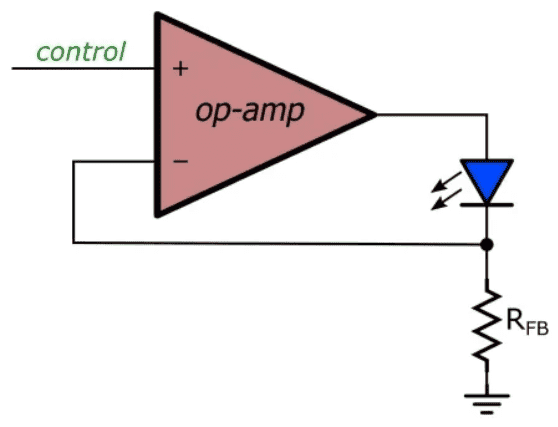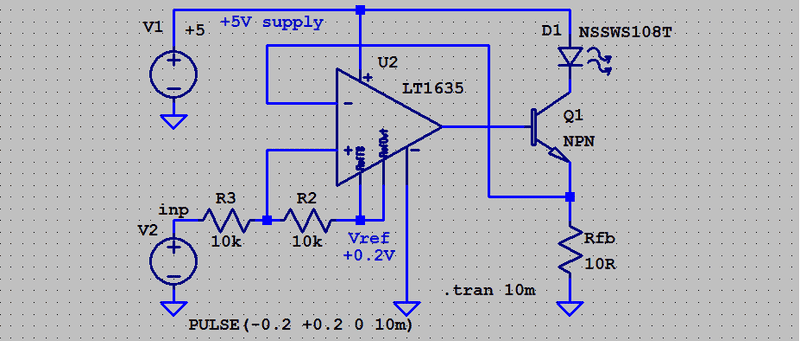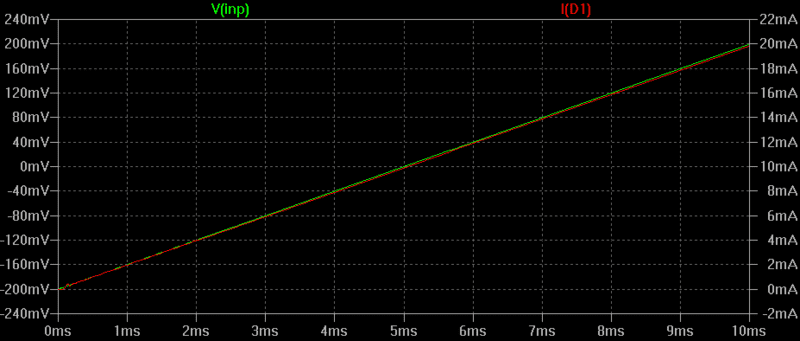# Proportional only controller in LED Driver using Op-Amp

• Engineering
PhysicsTruth
Homework Statement:
Construct a LED driver with ##K_P=10## for -0.2 - 0.2 V input. Correct it to 0-4V using an offset bias.
Relevant Equations:
Using the LED Driver Circuit equation -
##V_{control} = V_{LED/anode} - V_{Th}##
I have a proportional-only controller LED driver circuit -I need to fine-tune its ##K_P## to 10 for a significant input of -0.2 to 0.2 V in the steady state. In that case, should I use a potentiometer with 10:1 setting? The LED (actuator) can drive voltages between 0 to 4V, so I need to add a resistor bias offset voltage to the controller as well. Can someone help me in these? I know that I need to utilize the Thevenin circuit, so should I just make a resistor bias from my positive rail supply to ground? Cam someone help me out?

PhysicsTruth
Homework Statement:: Construct a LED driver with ##K_P=10## for -0.2 - 0.2 V input. Correct it to 0-4V using an offset bias.
Relevant Equations:: Using the LED Driver Circuit equation -
##V_{control} = V_{LED/anode} - V_{Th}##

I have a proportional-only controller LED driver circuit -
View attachment 291664
I need to fine-tune its ##K_P## to 10 for a significant input of -0.2 to 0.2 V in the steady state. In that case, should I use a potentiometer with 10:1 setting? The LED (actuator) can drive voltages between 0 to 4V, so I need to add a resistor bias offset voltage to the controller as well. Can someone help me in these? I know that I need to utilize the Thevenin circuit, so should I just make a resistor bias from my positive rail supply to ground? Cam someone help me out?
I guess I can use a potentiometer with 10:1 setting connected from the LED cathode and forming a voltage divider bias with the negative terminal of Op-Amp, and finally to ground. But, I'm having some problems in setting up the offset bias. How do I actually connect this bias to correct it to 0-4V for -0.2 to +0.2 V input, with ##K_P = 10## ?

I suspect you have not presented the original question as asked.
I cannot separate your assumptions from the question.

You should regulate the current through a LED, not the voltage across it.
The circuit you give is for a voltage controlled current sink, which is good.
The 4 V appears across Rfb, the current sense resistor, not the LED.

What is the power supply voltage? Is it sufficient for 4V + Vled ?
Do you know what maximum current will be needed by the LED?
Can the op-amp source that current or will you need something like an emitter follower?

Kp = 10; Ten what? mA/volt or volt/volt ?
Why not fix the maximum voltage across Rfb to 0.4 volt ?
Then design the required control voltage level shift.

PhysicsTruth
I suspect you have not presented the original question as asked.
I cannot separate your assumptions from the question.

You should regulate the current through a LED, not the voltage across it.
The circuit you give is for a voltage controlled current sink, which is good.
The 4 V appears across Rfb, the current sense resistor, not the LED.

What is the power supply voltage? Is it sufficient for 4V + Vled ?
Do you know what maximum current will be needed by the LED?
Can the op-amp source that current or will you need something like an emitter follower?

Kp = 10; Ten what? mA/volt or volt/volt ?
Why not fix the maximum voltage across Rfb to 0.4 volt ?
Then design the required control voltage level shift.
Kp is in terms of volt/volt, which is thus across Rfb. The Op-Amp is enough to source the current required. In order to set Kp at 10, I've used another resistor from the LED, which is approximately 9 times that of the feedback resistor. This does give me a output voltage of 10 times that of the input voltage through the output terminal of the Op-Amp, but this works for only positive voltage inputs. Negative voltage inputs seem to saturate the output. Can you suggest an alternative in this case?

Who says you must use Kp = 10 v/v ?
Again, what is the positive power supply voltage ?
Is there a negative supply ?

PhysicsTruth
Who says you must use Kp = 10 v/v ?
Again, what is the positive power supply voltage ?
Is theer a negative supply ?
Well, I'm supposed to build the proportional only controller such that for the input voltages of -0.2 to 0.2 V, I get an output around -2 to 2V from the output terminal. Now, the voltage required to drive the LED is around 0-4V, as I'm expected, but -2V wouldn't drive the LED. So, I need to create that offset bias to change it to 0 to 4V, so that the forward voltage for the LED would be 0 to 4V, and not -2 to 2V. Also, the positive rail supply is +8V, and there is a negative one with -8V supply.

PhysicsTruth
Who says you must use Kp = 10 v/v ?
Again, what is the positive power supply voltage ?
Is there a negative supply ?
Can you suggest what resistor values should I use for the voltage divider bias from the output of Op-Amp to the anode of LED, in order to shift the voltage level by 2V?

... Now, the voltage required to drive the LED is around 0-4V, ...
But the LED brightness is proportional to current, not linearly dependent on LED voltage.
LED voltage is dominated by the LED wavelength, plus a small increase with current.

Again, who says you must use Kp = 10 v/v ?
Is it in the original question, or is it just your assumption ?

Can you suggest what resistor values should I use for the voltage divider bias from the output of Op-Amp to the anode of LED, in order to shift the voltage level by 2V?
There must be an easier way.

PhysicsTruth
But the LED brightness is proportional to current, not linearly dependent on LED voltage.
LED voltage is dominated by the LED wavelength, plus a small increase with current.

Again, who says you must use Kp = 10 v/v ?
Is it in the original question, or is it just your assumption ?
I'm just instructed to obtain a Kp of 10, and since it deals with obtaining 0-4V input to the LED from -0.2 to 0.2V input, I assume it's a V/V Kp.

PhysicsTruth
There must be an easier way.
Well, I'm absolutely new to feedback control systems, and I've been asked to use a voltage divider for level shifting in this case. It would be great if you could help me out with the Thevenin equivalent.

Without current limiting, a forward voltage of 4 V will destroy most LEDs.
Likewise the reverse voltage maximum is 5 V, at very low current.
Your ±8 V supply is more than sufficient to destroy the LED.
You will need to know the maximum LED current before you can design the circuit.

PhysicsTruth
Without current limiting, a forward voltage of 4 V will destroy most LEDs.
Likewise the reverse voltage maximum is 5 V, at very low current.
Your ±8 V supply is more than sufficient to destroy the LED.
You will need to know the maximum LED current before you can design the circuit.
Well, I've a red LED, and it can support a maximum of 4V forward voltage with saturation current around 6mA. I say this because I've tested it out using a 4V reference.

Well, I've a red LED, and it can support a maximum of 4V forward voltage with saturation current around 6mA. I say this because I've tested it out using a 4V reference.
Then it is not a simple LED, it has a series resistance, Rs.
Red is a wavelength of about 640 nm.
The photon energy, in eV = 1239.84 / nm
So the voltage needed to make red photons is about v = 1.94 volts.
You measured current at 6 mA with total v = 4 V;
Vr = ( 4 - 1.94 ) = 2.06 V across the resistor Rs.
Ohms law; Rs = 2.06 / 0.006 = 343 ohms.
But there will be almost no light emitted until V ≥ 1.94 V.
Are you still sure you want to regulate LED voltage rather than control current ?

•PhysicsTruth
Gold Member
Well, I'm absolutely new to feedback control systems, and I've been asked to use a voltage divider for level shifting in this case. It would be great if you could help me out with the Thevenin equivalent.
A level shifter requires transistors. I believe you would need a pnp junction transistor to shift the negative output.

PhysicsTruth
A level shifter requires transistors. I believe you would need a pnp junction transistor to shift the negative output.
It does. But, using three resistors and a divider bias, we can shift the voltage level as well, if I'm not wrong. I'm unable to arrive at the values in that case.

PhysicsTruth
I've solved the problem regarding the saturation of the negative input voltages. If only someone could guide me how to design the voltage shift from -2 - 2 to 0 - 4 using three resistors and a divider bias

PhysicsTruth
Then it is not a simple LED, it has a series resistance, Rs.
Red is a wavelength of about 640 nm.
The photon energy, in eV = 1239.84 / nm
So the voltage needed to make red photons is about v = 1.94 volts.
You measured current at 6 mA with total v = 4 V;
Vr = ( 4 - 1.94 ) = 2.06 V across the resistor Rs.
Ohms law; Rs = 2.06 / 0.006 = 343 ohms.
But there will be almost no light emitted until V ≥ 1.94 V.
Are you still sure you want to regulate LED voltage rather than control current ?
Yes, I was doing it wrongly. I created a separate controller for the LED driver, and it's working fine now. Only, I need to get an output of 0-4V from the controller part (without the LED, it functions as a non-inverting amplifier), instead of a -2 V to 2 V output from -0.2 to 0.2V input. Can you give me a hint regarding how to setup the voltage divider with 3 resistors? I've been trying out configurations and have done some shifting, but can't obtain the 0-4 level-shifted output.

It is real bad practice to use a power supply rail as a voltage reference, mainly because it will add noise to the signal. Changing the power supply will require a recalibration.

The offset will require an accurate voltage or a current reference. You should be aware that there are a number of op-amps that have an 0.2 V buffered reference voltage, and can operate without a negative supply. The LM10 was the first example. There are many more now.•